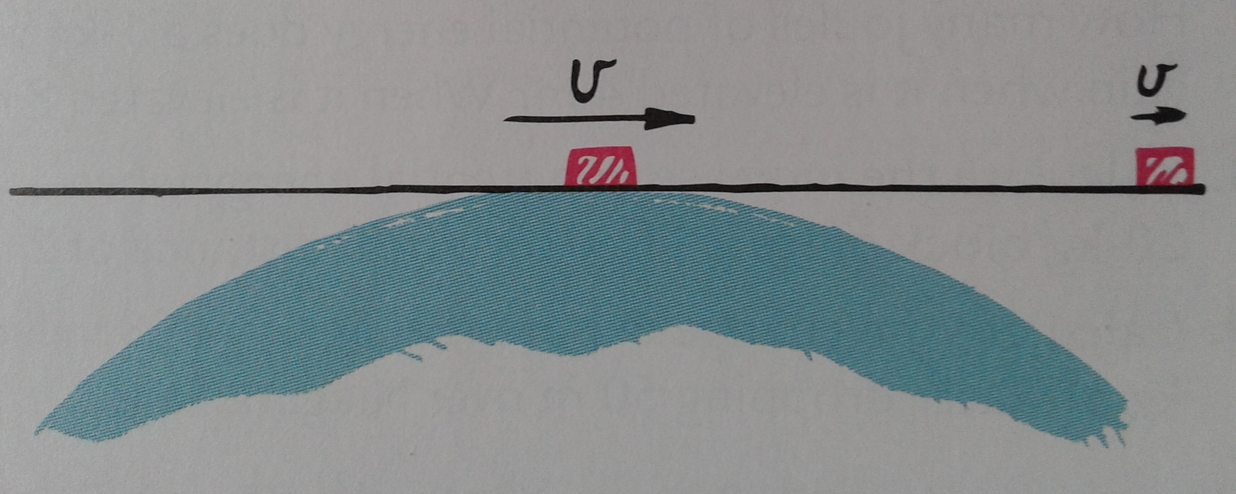# Conceptual Physics: IVSuppose an object is set sliding, with a speed less than escape velocity, on an infinite frictionless plane in contact with the surface of the Earth, as shown. Describe its motion. (Will it slide forever at a constant velocity? Will it slide to a stop? In what way will its energy changes be similar to that of a pendulum?)

$\large{\text{A}}$. The object will oscillate about the point of contact between the plane and the Earth's surface, sliding right, stopping, sliding to the left, stopping, and back again in a closed loop - just like a pendulum would if given an initial push.

$\large{\text{B}}$. Since the plane is frictionless, the object does not have to undergo any resistance to its horizontal motion unlike its terrestrial counterparts that have to move against the air resistance of the Earth's atmosphere. Hence, the escape velocity rules do not apply to the object, and the object will slide indefinitely - to infinity and beyond - just like a pendulum would swing in a circle inside a vacuum chamber.

$\large{\text{C}}$. The object will slide along the direction of its initial velocity, eventually to be pulled backwards until it passes its starting position. Then, the object will oscillate at the point somewhere between its starting position and its turning position - just like a pendulum would do starting with the highest velocity at the initial swing and then oscillating at the point between its starting position and its turning position.

$\large{\text{D}}$. The object will slide along the direction of its initial velocity, eventually to be pulled backward until it passes the point of contact between the plane and the Earth's surface. Then the object will again change directions, after sliding a lesser distance. This will keep happening until the object finally stops at that point in contact with Earth - just like a pendulum would eventually stop swinging and end up at its lowest point.

×